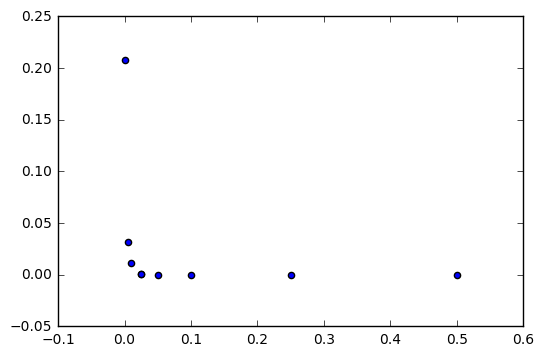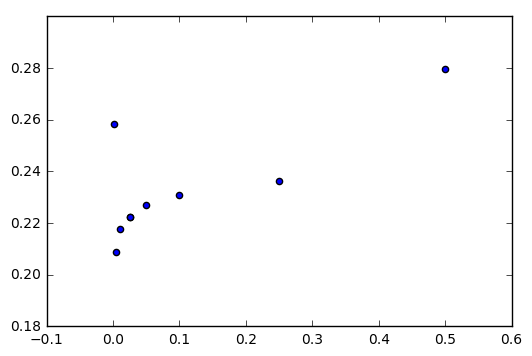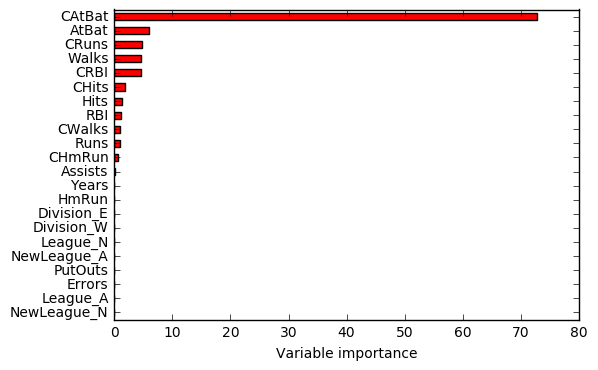# Exercise 8.10

import pandas as pd
import numpy as np
import matplotlib.pyplot as plt
from sklearn.metrics import mean_squared_error
from sklearn.linear_model import LinearRegression
from sklearn.linear_model import LassoCV
from sklearn.ensemble import BaggingRegressor

%matplotlib inline

df = pd.read_csv('../data/Hitters.csv', index_col=0)

df.head()

AtBat Hits HmRun Runs RBI Walks Years CAtBat CHits CHmRun CRuns CRBI CWalks League Division PutOuts Assists Errors Salary NewLeague
-Andy Allanson 293 66 1 30 29 14 1 293 66 1 30 29 14 A E 446 33 20 NaN A
-Alan Ashby 315 81 7 24 38 39 14 3449 835 69 321 414 375 N W 632 43 10 475.0 N
-Alvin Davis 479 130 18 66 72 76 3 1624 457 63 224 266 263 A W 880 82 14 480.0 A
-Andre Dawson 496 141 20 65 78 37 11 5628 1575 225 828 838 354 N E 200 11 3 500.0 N
-Andres Galarraga 321 87 10 39 42 30 2 396 101 12 48 46 33 N E 805 40 4 91.5 N
df = pd.get_dummies(df)

df.head()

AtBat Hits HmRun Runs RBI Walks Years CAtBat CHits CHmRun ... PutOuts Assists Errors Salary League_A League_N Division_E Division_W NewLeague_A NewLeague_N
-Andy Allanson 293 66 1 30 29 14 1 293 66 1 ... 446 33 20 NaN 1.0 0.0 1.0 0.0 1.0 0.0
-Alan Ashby 315 81 7 24 38 39 14 3449 835 69 ... 632 43 10 475.0 0.0 1.0 0.0 1.0 0.0 1.0
-Alvin Davis 479 130 18 66 72 76 3 1624 457 63 ... 880 82 14 480.0 1.0 0.0 0.0 1.0 1.0 0.0
-Andre Dawson 496 141 20 65 78 37 11 5628 1575 225 ... 200 11 3 500.0 0.0 1.0 1.0 0.0 0.0 1.0
-Andres Galarraga 321 87 10 39 42 30 2 396 101 12 ... 805 40 4 91.5 0.0 1.0 1.0 0.0 0.0 1.0

5 rows × 23 columns

# (a)

# Remove observations for whom the salary information is unknown.
df = df.dropna(subset=['Salary'])

# Log transform salaries
df['Salary'] = np.log(df['Salary'])


# (b)

# Create training and testing
# We don't use the train_test_split because we don't want to split data randomly.
X = df.drop(['Salary'], axis=1)
y = df['Salary']

X_train = X.ix[:200,:]
y_train = y.ix[:200]
X_test = X.ix[200:,:]
y_test = y.ix[200:]


# (c)

# Boosting with different shrinkage values
shrinkage_values = [.001, .025, .005, .01, .025, .05, .1, .25, .5]
mses = []
for i in shrinkage_values:
bst.fit(X_train, y_train)
mses.append(mean_squared_error(y_train, bst.predict(X_train)))

# Plot training set MSE for different shrinkage values
plt.scatter(shrinkage_values, mses)

<matplotlib.collections.PathCollection at 0xf98e0f0># (d)

# Boosting with different shrinkage values
shrinkage_values = [.001, .025, .005, .01, .025, .05, .1, .25, .5]
mses = []
for i in shrinkage_values:
bst.fit(X_train, y_train)
mses.append(mean_squared_error(y_test, bst.predict(X_test)))

# Plot training set MSE for different shrinkage values
plt.scatter(shrinkage_values, mses)

<matplotlib.collections.PathCollection at 0xf9f5240># Get minimum test MSE value
print('Minimum test MSE:', np.min(mses))

Minimum test MSE: 0.208753925111

# Index of the shrinkage_value that leads to the minimum test MSE
np.where(mses == np.min(mses))

(array(, dtype=int64),)


# (e)

# Linear regression
rgr = LinearRegression()
rgr.fit(X_train, y_train)

print('Minimum test MSE:', mean_squared_error(y_test, rgr.predict(X_test)))

Minimum test MSE: 0.491795937545

# Cross-validated lasso
lasso = LassoCV(cv=5)
lasso.fit(X_train, y_train)

print('Minimum test MSE:', mean_squared_error(y_test, lasso.predict(X_test)))

Minimum test MSE: 0.486586369603


The test MSE obtained using boosting is lower than the test MSE obtained using a linear regression or a lasso regularized regression. This means that, according to this error metric, boosting is the model with better predictive capacity.

# (f)

# Plot features importance to understand their importance.
bst = GradientBoostingRegressor(learning_rate=0.005)  # 0.005 is the learning_rate corresponding to the best test MSE
bst.fit(X_train, y_train)

feature_importance = bst.feature_importances_*100
rel_imp = pd.Series(feature_importance, index=X.columns).sort_values(inplace=False)
rel_imp.T.plot(kind='barh', color='r')
plt.xlabel('Variable importance')

<matplotlib.text.Text at 0xd682e10>According to the figure, the most important predictors seem to be: CAtBat, AtBat, CRuns, Walks and CRBI.

# (g)

# Fit bagging regressor
bagging = BaggingRegressor()
bagging.fit(X_train, y_train)

BaggingRegressor(base_estimator=None, bootstrap=True,
bootstrap_features=False, max_features=1.0, max_samples=1.0,
n_estimators=10, n_jobs=1, oob_score=False, random_state=None,
verbose=0, warm_start=False)

# Test MSE
print('Test MSE:', mean_squared_error(y_test, bagging.predict(X_test)))

Test MSE: 0.253358375264